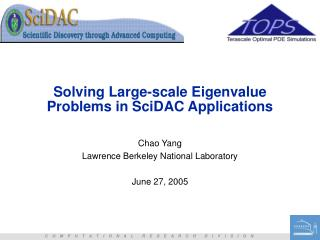# Solving Large-scale Eigenvalue Problems in SciDAC Applications - PowerPoint PPT PresentationDownload PresentationSolving Large-scale Eigenvalue Problems in SciDAC Applications

Solving Large-scale Eigenvalue Problems in SciDAC Applications
Download Presentation## Solving Large-scale Eigenvalue Problems in SciDAC Applications

- - - - - - - - - - - - - - - - - - - - - - - - - - - E N D - - - - - - - - - - - - - - - - - - - - - - - - - - -
##### Presentation Transcript

1. Solving Large-scale Eigenvalue Problems in SciDAC Applications Chao Yang Lawrence Berkeley National Laboratory June 27, 2005

2. People Involved • LBNL: • W. Gao, P. Husbands, X. S. Li, E. Ng, C. Yang (TOPS) • J. Meza, L. W. Wang, C. Yang (Nano-science) • SLAC: • L. Lee, K. Ko • Stanford: • G. Golub • UC-Davis • Z. Bai

3. SciDAC Applications • Accelerator Modeling • Nano-science

4. Algorithms • Krylov Subspace Method • Alternatives • Optimization based approach • non-linear solver based approach • Multi-level Sub-structuring • Non-linear Eigenvalue Problems • Structure preserving methods • Optimization based method

5. Krylov Subspace Method • Widely used, relatively well understood (Polynomial approximation theory): • Convergence of KSM: • Well separated, large eigenvalues converge rapidly • the starting vector

6. Acceleration Techniques • Implicit Restart • Spectral transformation ARPACK filter out unwanted spectral components from v0

7. Using KSM in accelerator modeling • the spectrum of the problem • Example: H60VG3 structure, linear element, N=30M, nnz=484M • 1024 CPUs, 738GB • Ordering time: 4143s • Numerical Factorization: 133s • Total: 5068s for 12 eigenvalues • Software: PARPACK (implicit restart) + SuperLU, WSMP (spectral transformation)

8. Limitations of the KSM • High degree polynomial needed for computing small clustered eigenvalues • many matrix vector multiplications • Spectral transformation can be expensive • memory limitation • scalability • Not easy to introduce a preconditioner • eigenvectors of P-1A are different from eigenvectors of A

9. Alternative algorithms • Optimization based approach • Minimizing Rayleigh Quotient • Minimizing Residual (Wood & Zunger 85, Jia 97) • Nonlinear equation solver based approach (Jacobi-Davidson) • Newton correction • Preconditioner • stopping criteria for the inner iteration (Notay 2002, Stathopoulos 2005) Allows us to solve problems with more than 90M DOF

10. Multi-level Sub-structuring (for computing many eigenpairs) • Domain Decomposition concept • Multi-level extension of the Component Mode Synthesis (CMS) method (Bennighof 92) • Decomposition can be done algebraically (Lehoucq & Bennighof 2002) • Success story in structure engineering.... • Error analysis • Extend to accelerator modeling

11. Matrix Partition Block elimination Sub-structure calculation (mode selection) Subspace assembling Single-level Sub-structuring

12. Mode Selection

13. Implementation & cost • Cost: • Flops: more than a single sparse Cholesky factorization • Storage: Block Cholesky factor + Projected matrix + some other stuff • NO triangular solves (involving the original K and M), NO orthogonalization attractive when: 1) the problem is large enough 2) a large number of eigenvalues are needed

14. AMLS vs. Shift-invert Lanczos (SIL) DOF=65K, 3 levels of partition

15. Waveguide BC Waveguide BC Open Cavity Waveguide BC With Cavity with External Coupling • Vector wave equation with waveguide boundary conditions can be modeled by a non-linear eigenvalue problem

16. Quadratic Eigenvalue Problem • Consider only one mode propagating in the waveguides • Algorithms • Linearize then solve by KSM (does not preserve the structure of the problem) • Second Order Arnoldi Iteration (Bai & Su 2005) project the QEP into 2nd order Krylov Subspace

17. Second-Order Krylov Space (Bai)

18. SOAR is faster and more accurate (than linearization) • Accelerating cavity model for international linear collider (ILC) • 9-cell superconducting cavity coupled to one input coupler and two Higher-Order-Mode couplers. • NDOFs=3.2million, NCPUs=768, Memory=300GB • 18 eigenpairs in 2634 seconds (linearization took more than 1 hour)

19. wave function n – real space grid size, e.g. 323~32000 k – number of occupied states, 1~10% of n Charge density Electronic Structure Calculation Etotal(X) = Ekinetic + Eionic + EHartree + Exc • Ekinetic = • Eionic = • EHartree= • Exc =

20. Non-linear Eigenvalue Problem • Total energy minimization • KKT condition

21. The Self Consistent Field Iteration • Input: initial guess and • Output: • Major steps • For i=1,2,…,until converged • Form • Compute k smallest eigpairs of

22. Direct Constrained Minimization (DCM) • For i=1,2,… until convergence • Form • Compute • If (i>1) then • set • else • set • Solve • If (i>1) then • set • else • set

23. DCM vs. SCF • Atomic system: SiH4 • Discretization: spectral method with plane wave basis: n=323 in real space, N=2103 (# of basis functions) in frequency space • Number of occupied states: k = 4 • PETOT version of SCF uses 10 PCG steps (inner iterations) per outer iteration • DCM: 3 inner iterations

24. Concluding Remarks • Krylov Subspace Method (with appropriate acceleration strategies) continues to play an important role in solving SciDAC eigenvalue problems • Steady progress has been made in alternative approaches that can make better use of preconditioners • Multi-level sub-structuring is promising for computing many eigenpairs • Significant progress made in solving QEP • Non-linear eigenvalue problems remain challenging